\$B:#>,\$N0lGU(B

\$BKhF|!":#>,\$N0lGU\$r>e\$2\$F\$\$\$^\$9!#(B
\$B:#>,0{\$`\$b\$N\$K:\$\$C\$?\$i!";29M\$K\$7\$F\$_\$F\$/\$@\$5\$\$!#(B

2021\$BG/(B2\$B7n(B

 1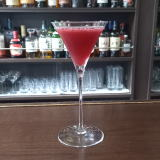(IZ/D^%=C0<^(B 2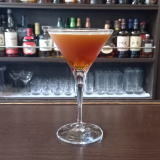(IRD[N_X@]\$B-b(B1 3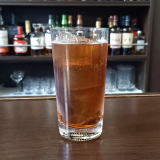(IO2]D^%2Z0;^0(B 4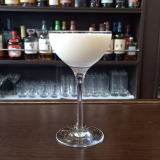(IR[C^(0(B 5(I70Y%2]M_X1Y(B 6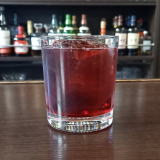(IN_]K_4%C^2<^0(B 7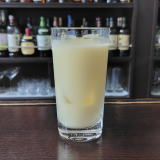(IO]:^T]%W/<0(B 8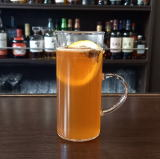(I8^[/8^(B 9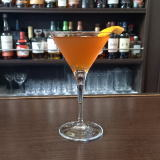(IJ_DX<1(B 10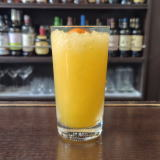(I5Z]<^%8W/<-(B 11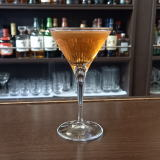(IX5HY(B 12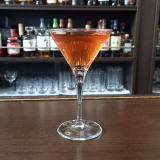(IEN_Z5](B 13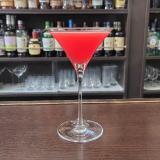(IS0F]8^%[0=^(B 14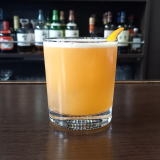(IL_Z2R2D(B 15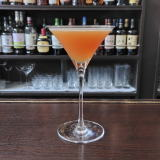(I5X4]@Y(B 16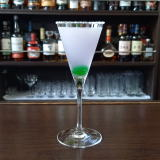(IC(]60M^Y(B 17(IJ_2Z0B(B 18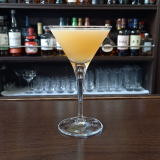(I@26^0=%12(B 19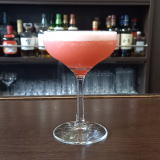(IK_]8%J_0Y(B 20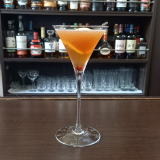(IJ_]A.%K^W(B 21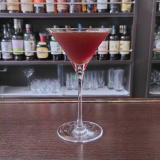(I=[0<^]%68CY(B 22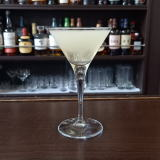(I=L_X]8^@2Q%OC(0F(B 23(IL_0=%WK_40Y(B 24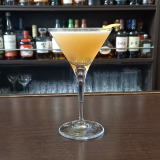(I5YZ1F1](B 25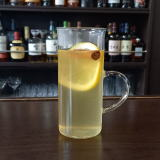(IN/D%D^WQ(B 26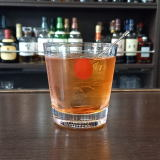(IC(0A,0=^%M_/D(B 27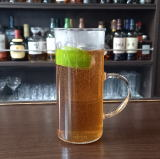(IN/D%S=:P-0Y(B 28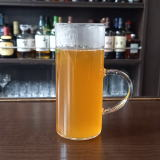(IN/D%SK0D(B